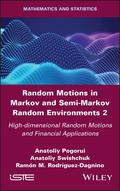John Wiley & Sons Random Motions in Markov and Semi-Markov Random Environments 2This book is the second of two volumes on random motions in Markov and semi-Markov random environmen.. Product #: 978-1-78630-706-4 Regular price: \$142.06 \$142.06 In Stock

# Random Motions in Markov and Semi-Markov Random Environments 2

## High-dimensional Random Motions and Financial Applications1. Edition February 2021
224 Pages, Hardcover
Wiley & Sons Ltd

ISBN: 978-1-78630-706-4
John Wiley & Sons

### Price: 152,00 €

Price incl. VAT, excl. Shipping

This book is the second of two volumes on random motions in Markov and semi-Markov random environments. This second volume focuses on high-dimensional random motions. This volume consists of two parts. The first expands many of the results found in Volume 1 to higher dimensions. It presents new results on the random motion of the realistic three-dimensional case, which has so far been barely mentioned in the literature, and deals with the interaction of particles in Markov and semi-Markov media, which has, in contrast, been a topic of intense study.

The second part contains applications of Markov and semi-Markov motions in mathematical finance. It includes applications of telegraph processes in modeling stock price dynamics and investigates the pricing of variance, volatility, covariance and correlation swaps with Markov volatility and the same pricing swaps with semi-Markov volatilities.

Preface ix

Acknowledgments xiii

Introduction xv

Part 1. Higher-dimensional Random Motions and Interactive Particles 1

Chapter 1. Random Motions in Higher Dimensions 3

1.1. Random motion at finite speed with semi-Markov switching directions process 5

1.1.1. Erlang-K-distributed direction alternations 7

1.1.2. Some properties of the random walk in a semi-Markov environment and its characteristic function 15

1.2. Random motion with uniformly distributed directions and random velocity 17

1.2.1. Renewal equation for the characteristic function of isotropic motion with random velocity in a semi-Markov media 17

1.2.2. One-dimensional case 20

1.2.3. Two-dimensional case 23

1.2.4. Three-dimensional case 23

1.2.5. Four-dimensional case 31

1.3. The distribution of random motion at non-constant velocity in semi-Markov media 32

1.3.1. Renewal equation for the characteristic function 34

1.3.2. Two-dimensional case 35

1.3.3. Three-dimensional case 37

1.3.4. Four-dimensional case 40

1.4. Goldstein-Kac telegraph equations and random flights in higher dimensions 43

1.4.1. Preliminaries about our modeling approach 45

1.4.2. Two-dimensional case 48

1.4.3. Three-dimensional case 51

1.4.4. Five-dimensional case 59

1.5. The jump telegraph process in R^n 62

1.5.1. The jump telegraph process in R³ 63

1.5.2. Conclusions and final remarks 64

Chapter 2. System of Interactive Particles with Markov and Semi-Markov Switching 67

2.1. Description of the Markov model 68

2.1.1. Distribution of the first meeting time of two telegraph processes 69

2.1.2. Estimate of the number of particle collisions 74

2.1.3. Free path times of a family of particles 76

2.1.4. Estimation of the number of particle collisions for systems with boundaries 78

2.1.5. Estimation of the number of particle collisions for systems without boundaries 83

2.2. Interaction of particles governed by generalized integrated telegraph processes: a semi-Markov case 87

2.2.1. Laplace transform of the distribution of the first collision of two particles 88

2.2.2. Semi-Markov case 91

2.2.3. Distribution of the first collision of two particles with finite expectation 95

Part 2. Financial Applications 99

Chapter 3. Asymptotic Estimation for Application of the Telegraph Process as an Alternative to the Diffusion Process in the Black-Scholes Formula 101

3.1. Asymptotic expansion for the singularly perturbed random evolution in Markov media in the case of disbalance 101

3.2. Application: Black-Scholes formula 106

Chapter 4. Variance, Volatility, Covariance and Correlation Swaps for Financial Markets with Markov-modulated Volatilities 111

4.1. Volatility derivatives 111

4.1.1. Types of volatilities 111

4.1.2. Models for volatilities 113

4.1.3. Variance and volatility swaps 115

4.1.4. Covariance and correlation swaps 116

4.1.5. A brief literature review 117

4.2. Martingale representation of a Markov process 118

4.3. Variance and volatility swaps for financial markets with Markov-modulated stochastic volatilities 122

4.3.1. Pricing variance swaps 124

4.3.2. Pricing volatility swaps 124

4.4. Covariance and correlation swaps for two risky assets for financial markets with Markov-modulated stochastic volatilities 128

4.4.1. Pricing covariance swaps 128

4.4.2. Pricing correlation swaps 130

4.4.3. Correlation swap made simple 130

4.5. Example: variance, volatility, covariance and correlation swaps for stochastic volatility driven by two state continuous Markov chain 132

4.6. Numerical example 134

4.6.1. S&P 500: variance and volatility swaps 134

4.6.2. S&P 500 and NASDAQ-100: covariance and correlation swaps 135

4.7. Appendix 1 138

4.7.1. Correlation swaps: first-order correction 138

Chapter 5. Modeling and Pricing of Variance, Volatility, Covariance and Correlation Swaps for Financial Markets with Semi-Markov Volatilities 143

5.1. Introduction 143

5.2. Martingale representation of semi-Markov processes 148

5.3. Variance and volatility swaps for financial markets with semi-Markov stochastic volatilities 151

5.3.1. Pricing of variance swaps 153

5.3.2. Pricing of volatility swaps 155

5.3.3. Numerical evaluation of variance and volatility swaps with semi-Markov volatility 158

5.4. Covariance and correlation swaps for two risky assets in financial markets with semi-Markov stochastic volatilities 159

5.4.1. Pricing of covariance swaps 160

5.4.2. Pricing of correlation swaps 162

5.5. Numerical evaluation of covariance and correlation swaps with semi-Markov stochastic volatility 164

5.6. Appendices 165

5.6.1. Appendix 1. Realized correlation: first-order correction 165

5.6.2. Appendix 2. Discussions of some extensions 169

References 177

Index 191

Summary of Volume 1 193
Anatoliy Pogorui?s main research interests include probability, stochastic processes, mathematical modeling of an ideal gas using multi-dimensional random motions and the interaction of telegraph particles in semi-Markov environments and the application of random evolutions in the reliability theory of storage systems.

Anatoliy Swishchuk is Professor of mathematical finance at the University of Calgary, Canada. His research areas include financial mathematics, random evolutions and their applications, stochastic calculus and biomathematics.

Ramon M. Rodriguez-Dagnino has investigated applied probability aimed at modeling systems with stochastic behavior, random motions in wireless networks, video trace modeling and prediction, information source characterization, performance analysis of networks with heavytail traffic, generalized Gaussian estimation and spectral analysis.